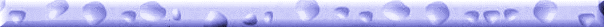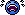Examples page

\r\n"; \$DaCounter = 0; while (\$row = mysql_fetch_row(\$dbresult)) { \$DaTitle = \$row; \$DaLink = \$row; \$DaURL = \$row; \$DaBadlink = \$row; if (\$DaCounter == 5) { print "\r\n"; print "\r\n"; \$DaCounter = 0; } \$DaCounter = \$DaCounter + 1; print "\r\n"; } while (\$DaCounter <= 5) { if (\$DaCounter == 0) { print "\r\n"; } if (\$DaCounter == 1) { print "\r\n"; } if (\$DaCounter == 2) { print "\r\n"; } if (\$DaCounter == 3) { print "\r\n"; } if (\$DaCounter == 4) { print "\r\n"; } \$DaCounter = \$DaCounter + 1; } print "

[Bad link]\r\n"; } if (\$DaURL != "" && \$DaURL != "nopic") { print "\r\n"; } print "

Wayback Machine mirror\r\n"; print "

Thisspaceintentionallyleftblank
\r\n"; } } // ------ Function ends -------- // We do it this way so I don't have to type as much... besides... functions are cool ?>

 Hall Of Fame!Cocktail CabinetsDriving and other sit-down CabinetsProjects that don't fit anywhere else!JukeboxesBartop/CountertopMiscellaneous HacksDead links(Can you help fix one of these?)